## 30 Apr 2016

### Agler (5.2) Symbolic Logic: Syntax, Semantics, and Proof, "Premises and the Goal Proposition", summary

by Corry Shores
[Search Blog Here. Index-tags are found on the bottom of the left column.]

[The following is summary. Boldface (except for metavariables) and bracketed commentary are my own. Please forgive my typos, as proofreading is incomplete. I highly recommend Agler’s excellent book. It is one of the best introductions to logic I have come across.]

Summary of

David W. Agler

Symbolic Logic: Syntax, Semantics, and Proof

Ch.5: Propositional Logic Derivations

5.2 Premises and the Goal Proposition

Brief summary:

In a proof, we can for convenience write the goal proposition, which is also the conclusion, next to the ‘P’ for the final premise, in the justification column.

Summary

In our proof, we have propositions that serve as the premises. They are noted as such in the justification column with the letter P. Suppose we wanted to make a proof for the following argument:

A→B, B→C ⊢ C→D

[To make the argument more obvious, I will change the conclusion to A→C in the diagrams. So instead, the argument would be:

A→B, B→C ⊢ A→C

] We would set up the premises like this: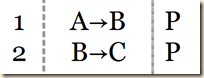Our proof would like to arrive upon the conclusion, which in this case is A→C. We call this the goal proposition, and for convenience we can write it beside the ‘P’ of the last premise.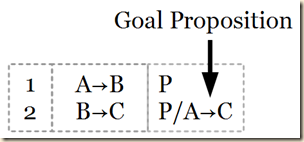(Agler 163)

Agler, David. Symbolic Logic: Syntax, Semantics, and Proof. New York: Rowman & Littlefield, 2013.

### Agler (5.1) Symbolic Logic: Syntax, Semantics, and Proof, "Proof Construction", summary

by Corry Shores
[Search Blog Here. Index-tags are found on the bottom of the left column.]

[The following is summary. Boldface (except for metavariables) and bracketed commentary are my own. Please forgive my typos, as proofreading is incomplete. I highly recommend Agler’s excellent book. It is one of the best introductions to logic I have come across.]

Summary of

David W. Agler

Symbolic Logic: Syntax, Semantics, and Proof

Ch.5: Propositional Logic Derivations

5.1 Proof Construction

Brief summary:

We can use a natural deduction system in order to make proofs for the conclusions of arguments. Such a system provides derivation rules, which allow us to move forward in a proof by obtaining new propositions on the basis of previously established ones. When a conclusion is provable by means of such a system, we say that the conclusion is a syntactical consequence of, or that that it is syntactically entailed by, the premises. And to signify this we use the turnstile symbol, as for example in this argument: R∨S, ¬S ⊢ R.  If we have simply ⊢P, that means there is a proof of P or that P is a theorem. In our proofs, there are three columns. The left column gives the line number. The central column shows the proposition. And the right column gives the justification, which is either that the proposition is a premise, in which case we write ‘P’, or that it is derived from other propositions, in which case we list the line numbers of those other propositions and write the abbreviation for the derivation rule that was used.

Summary

Ch.5: Propositional Logic Derivations

In chapters 3 and 4, we used truth tables and truth trees to test for certain semantic properties of singular propositions (tautology, contradiction, contingency), sets of propositions (consistency, inconsistency, equivalence), and arguments (validity). While these tests using tables and trees are useful for these purposes, “These tests do not, however, correspond to the reasoning that takes place in daily life” (161). So in this chapter we will examine a system of natural deduction. We learn rules for deriving new propositions from given ones, and all of this is modeled on normal human reasoning.

A natural deduction system is a set of derivational rules (general steps of reasoning) that mirror everyday reasoning in certain noteworthy ways. The particular system will be called a system of propositional derivations, or PD for short. Unlike truth tables and truth trees, PD is a system of syntactic rules insofar as they are formulated on the basis of the structure of everyday reasoning.

(161)

After we learn the basics of PD, we then examine more advanced systems and study certain strategies, which will not be like normal everyday reasoning, but it can still make reasoning more efficient (161).

5.1 Proof Construction

The purpose of learning this system of natural deduction is to solve proofs. Agler defines proof in the following way.

Proof: A proof is a finite sequence of well-formed formulas (or propositions), each of which is either a premise, an assumption, or the result of preceding formulas and a derivation rule.

(Agler 161)

Agler then works through the definition part-by-part. “A proof is a finite sequence of well-formed formulas...” It is finite, meaning that it will terminate as some point. And it is made of wffs, so they should follow the formation rules we saw in section 2.3.3. The end point we call the conclusion. Continuing: “...well-formed formulas (or propositions), each of which is either a premise, an assumption, or the result of preceding formulas and a derivation rule.” Agler says that

A derivation rule is an explicitly stated rule of PD that allows for moving forward in a proof. For example, if there is a derivation rule that says whenever you have ‘P’ and ‘Q,’ you can move a step forward in the proof to the proposition ‘P∧Q,’ then this derivation rule would justify (or legitimate) ‘P∧Q’ in a proof involving ‘P’ and ‘Q.’

(Agler 162)

In prior chapter we used the single turnstile symbol ⊢ simply to indicate that we were dealing with a set of propositions constituting an argument. The ⊢ came right before the proposition that served as the conclusion. Now we give ⊢ a more specific sense. It means syntactical entailment. [The idea seems to be the following. Suppose we have a set of propositions with the ⊢ symbol coming before the last of them, which is the conclusion of the argument. The ⊢ means that a proof can be made on the basis of the premises, making the conclusion true. Let me quote.]

In this chapter, ‘⊢’ takes on a more precise meaning, namely, that of syntactic entailment. Thus,

PR R

means that there is a proof of ‘R’ from ‘PR,’ or ‘R’ is a syntactic consequence of ‘PR.’ In the above example, ‘R’ is the conclusion of the proof, whereas ‘PR’ are its premises. In addition,

R

means that there is a proof of ‘R,’ or that ‘R’ is a theorem. In the above example, ‘R’ is the conclusion of a proof with no premises.

The simple idea of a proof then is one that begins with a set of premises and in which each subsequent step in the proof is justified by a specified rule

(Agler 162)

There are three main columns in a proof. On the far left we give the line number. In the center we have the propositions. And on the right we have the justification or an indication of the proposition being a premise. So consider the argument:

R∨S, ¬S ⊢ R

Let us set up the premises first.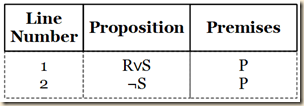As we continue with making the proof, we will use derivation rules, which will be indicated as justifications, along with the lines they are operating on.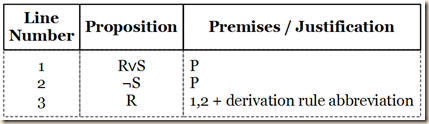Our proof is complete as soon as we have arrived upon the conclusion. In the above example, we concluded the proof in line 3, since R was the conclusion of the argument: R∨S, ¬S ⊢ R. (Agler 163)

Agler, David. Symbolic Logic: Syntax, Semantics, and Proof. New York: Rowman & Littlefield, 2013.

### Agler (4.6) Symbolic Logic: Syntax, Semantics, and Proof, "Logical Properties of Truth Trees", summary

by Corry Shores
[Search Blog Here. Index-tags are found on the bottom of the left column.]

[The following is summary. Boldface (except for metavariables) and bracketed commentary are my own. Please forgive my typos, as proofreading is incomplete. I highly recommend Agler’s excellent book. It is one of the best introductions to logic I have come across.]

Summary of

David W. Agler

Symbolic Logic: Syntax, Semantics, and Proof

Ch.4: Truth Trees

4.6 Logical Properties of Truth Trees

Brief summary:

By using truth trees we can test for logical properties of individual propositions, sets of propositions, and arguments.

Consistency: a set of propositions is consistent if their truth tree is completed open, that is to say, if we find at least one open branch.

Inconsistency: a set of propositions is inconsistent when their truth tree is closed, that is to say, when all its branches are closed.

Tautology: a singular proposition is a tautology when the truth tree for its negation is closed.

Contradiction: a singular proposition is a contradiction, when its truth tree is closed.

Contingency: supposing that we have already determined that a singular proposition is not a tautology, then it is a contingency rather than a contradiction, if its truth tree is open. In other words, if the neither the tree for the proposition nor for its negation is closed, then it is a contingency.

Equivalence: two propositions are equivalent if the tree for their negated biconditional combination is closed.

Validity: an argument is valid if the set of propositions made of the premises and the negated conclusion makes a closed tree.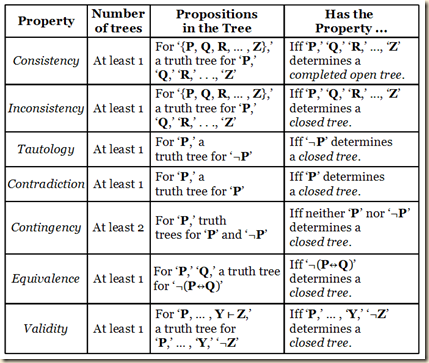(Agler 159)

Summary

4.6 Logical Properties of Truth Trees

We have been learning how to decompose truth trees. In this process, we come to determine whether or not a set of propositions creates a completed open tree (when there is at least one open branch) or a closed tree (when all branches close). These two structural features will serve as indicators for particular logical properties that these propositions can have.

4.6.1 Semantic Analysis of Truth Trees

Recall from chapter 3 how we used truth tables to determine whether propositions, sets of propositions, and arguments have certain logical properties. [In section 3.3 we learned how to determine if a singular statement is  a tautology, a contradiction, or a contingency. It is a tautology if it is true under all value assignments; for example,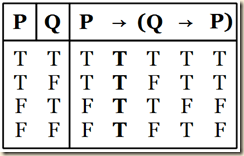it is a contradiction if it is false under all value assignments; for example,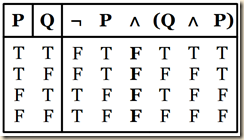and it is a contingency if it is either true or false, depending on what the value assignments are; for example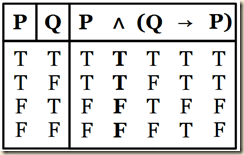In section 3.4 we examined sets of propositions just as a group, and not as if there involved some inferred conclusion. We saw how to determine whether or not pairs are equivalent and whether or not sets are consistent. If the truth table for a set of propositions shows them each to have identical truth values for any truth assignments, then they are logically equivalent; for example,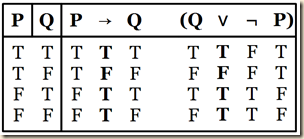And they are not equivalent otherwise. We can also test two propositions for equivalence by placing them into a biconditional relationship and seeing if it is a tautology, that is to say, seeing if all possible assignments produce the value true for the biconditional.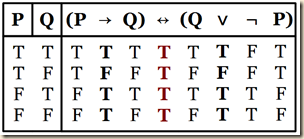A set of propositions are consistent if there is at least one value assignment that makes them all true. So on the truth table, we look for at least one line where all the propositions in question have the value true, and that tells us they are consistent; for example,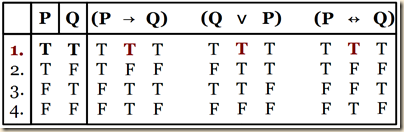The propositions are inconsistent if no truth value assignment makes all the propositions jointly true; for example,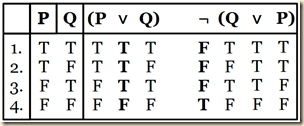We can also test for inconsistency by combining a pair of propositions with a conjunction. If the new proposition is a contradiction, then the original propositions are inconsistent; for example,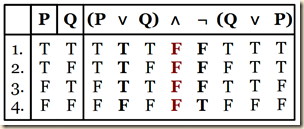In section 3.6 we used truth-tables to test whether or not an argument, that is a set of propositions including among them a conclusion that was inferred from the others, is valid or invalid. There were a number of ways for testing for validity. (a) We look for a row where all the premises are true and the conclusion false. If there is such a row, it is invalid.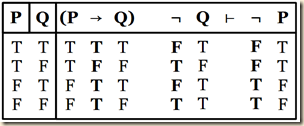And it is valid otherwise.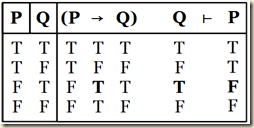(b) We convert the argument into a set of propositions with the premises left intact but the conclusion is negated. If that set of propositions is inconsistent, that is, if there is no row where they are all true, then the original argument is valid. However, if that set is consistent, that is, if there is at least one row where they are all true, then the original argument is invalid. So consider for example the argument:

P → Q, P ⊢ Q

Let us turn it into a set of propositions, with the conclusion now in a negated form.

{P→Q, P, ¬Q}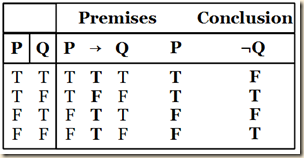As we can see from the table, there is no row where all the propositions are true. Thus the set of propositions {P→Q, P, ¬Q} is inconsistent, which means that the argument “P → Q, P ⊢ Q” is valid.] We will now use truth trees to make the same determinations of logical properties that we made previously with truth tables. [Recall from section 4.2 that a branch is a closed branch when it contains a proposition ‘P’ and its literal negation ‘¬P,’ and we place an X marking at the bottom of the branch. A branch is a completed open branch when it is completely decomposed and yet  does not contain such a contradiction. At its  bottom we place an 0 marking.] Whenever we have an open branch on a truth tree, all the atomic propositions along that open branch indicates truth assignments that make all the propositions true. If the atomic formula is negated, then we assign that propositions (in its non-negated form) the value false. And it is true if it is not negated. [Agler says it better, so let me quote him:]

Recall that decomposing a proposition ‘P’ consists of representing the conditions under which ‘P’ is true by stacking, branching, or branching and stacking. In the case of a completed open branch, it is possible to recover a set of valuations of the propositional letters. To see this more clearly, consider the truth tree for the following set of propositions: ‘{R∧¬M, R∧(W∧¬M)}.’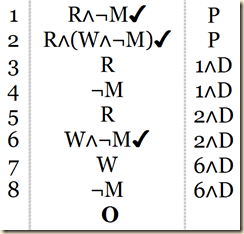Notice that there is one completed open branch of propositions consisting of ‘¬M,’ ‘W,’ ‘R.’ We can interpret this branch to recover a set of valuations of the propositional letters in a way that ‘R∧¬M’ and ‘R∧(W∧¬M)’ are jointly true. The method by which this is done proceeds in two steps. First, for every completed open branch, identify any atomic propositions and any negated atomic propositions. In the tree above, there is one completed open branch, consisting of ‘R,’ ‘W,’ and ‘¬M.’ Second, | assign a value of true to atomic propositions and false to negated propositional letters. This procedure determines a valuation set. In the case of ‘R,’ ‘W,’ and ‘¬M,’ we assign their truth values as follows:

v(R) = T

v(W) = T

v(M) = F

(Agler 138-139)

Agler then has us consider a tree for these propositions:

R∧W

M∨¬W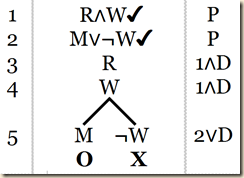[Recall from section 4.2 that a tree is a completed open tree only if it has at least one completed open branch.] Only the left branch will give us a consistent set of truth valuations that can make all the original propositions true. Along that left branch we have atomic propositions M, W, and R. Thus these are our value assignments for making the propositions jointly true:

v(M) = T

v(W) = T

v(R) = T

(Agler 139)

Now let us decompose the following propositions.

M→P

¬(P∨Q)

(R∨S)∧¬P

(Agler 140)

First we will place them as propositions.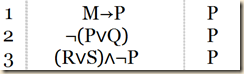We should begin with stacking rules first. We have a conjunction in line 3, so let us decompose that one now.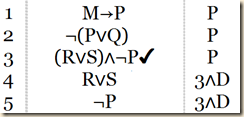We also would use a stacking rule for the negated disjunction in line 2. So we do that next.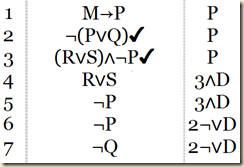This leaves us with two branching propositions, M→P in line 1 and R∨S in line 4. If we decompose R∨S , then we get R or S. But this creates no contradictions and thus has no branch closings. However, if we decompose M→P, we get ¬M or P. This creates a contradiction with ¬P from above, so let us do that one next.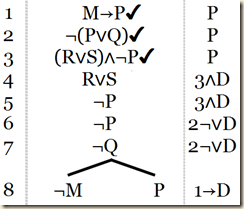As we can see, it is the right branch that now closes.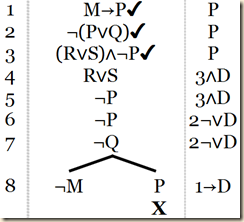That leaves us with R∨S.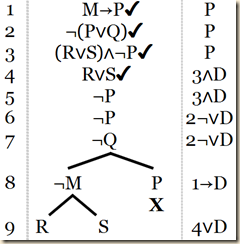As we noted before, it creates no contradictions, so we note that both these branches are open.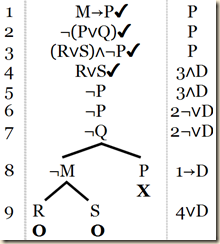We see that we have two open branches. In the leftmost branch we have these atomic formulas: ‘¬M,’ ‘¬P,’ and ‘¬Q,’. Let us give them their proper truth valuations. But we also notice that S is in the original propositions, even though it is not decomposed in this leftmost branch. So we do not have a determinate value for it yet.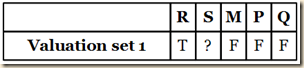What this means is that for this branch of assignments, it does not matter whether it is true or false. If it is true, then still it together with the other assignments will make all the propositions true. Or if it is false, it nonetheless will, taken together with the other determined valuations, make the original propositions all true. So we can fork this into two separate value assignments, one for each situation of S’s value.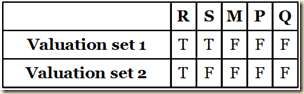Now let us look at the other open branch. Tracing up the path, we get these valuations: v(M) = F, v(P) = F, and v(Q) = F. But since we do not have R in this branch, we should make two valuations, one for each possible value for R.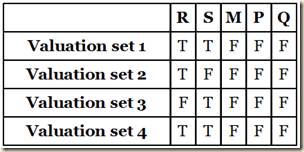But notice that valuation set 4 is identical to set 1. Since it is superfluous, we can eliminate it.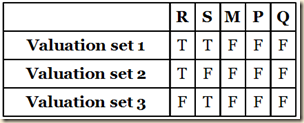Thus, using the truth-tree method, we have been able to determine that ‘’M→P,’ ‘¬(P∨Q),’ and ‘(R∨S)∧¬P’ are jointly true under three different possible valuations.

(Agler 141)

4.6.2 Consistency

We now learn how to use truth trees to determine whether or not a set of propositions are consistent. We recall from section 3.4 that we used truth tables to see if there was some truth-value assignment where all the propositions were truth, that is, if there was some row of the truth table were all the propositions evaluate as true. We made this table for the set of propositions: ‘P→Q,’ ‘Q∨P,’ and ‘P↔Q.’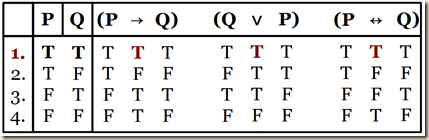We can see that row 1 shows the consistency of these propositions. The truth value assignment that makes them all true is: v(P) = T and v(Q) = T. Agler then defines consistency and inconsistency for truth trees in the following way:

Consistent: A set of propositions ‘{P, Q, R, ... , Z}’ is consistent if and only if there is at least one valuation where ‘P,’ ‘Q,’ ‘R,’ ... , ‘Z’ are true. A truth tree shows that ‘{P, Q, R, ... , Z}’ is consistent if and only if a complete tree of the stack of ‘P,’ ‘Q,’ ‘R,’ ... , ‘Z’ determines a completed open tree, that is, if there is at least one completed open branch.

Inconsistent: A set of propositions ‘{P, Q, R, ... , Z}’ is logically inconsistent if and only if there is no valuation where ‘P,’ ‘Q,’ ‘R,’ ... , ‘Z’ are jointly true. A truth tree shows that ‘{P, Q, R, ... , Z}’ is inconsistent if and only if a tree of the stack of ‘P,’ ‘Q,’ ‘R,’ ... , ‘Z’ is a closed tree, that is, if all branches close.

(Agler 143)

So to determine if a set of propositions is consistent, we first set them in the tree as propositions, stacking them with each getting its own line. Then we decompose it. Agler again uses the set: ‘{R∧¬M, R∧(W∧¬M)}.We decompose the tree until either it closes entirely or until there is at least one completed open branch. Then we analyze the tree. The one above has at least one completed open branch. This means that “there is at least one valuation set that would make the propositions in the set jointly true” (Agler 144). Thus it is consistent.

The second example we consider is the set: {R∧W, M∨¬W}. We saw it before:It is a completed open tree, because it “contains at least one completed open branch.” Thus that set of propositions is consistent (144).

For a final example, we consider this set of propositions [from section 4.5]

P→Q

T→[(Q∨R)∨(¬S∨M)]

(P∨Q)∨R

(P→M)∨[W↔(¬S∨S)]

¬(¬P∨Q)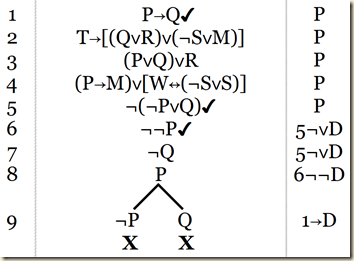As Agler notes, “The above tree is complete and closed. It is thus inconsistent because there is not a completed open branch, for all of the branches are closed” (144).

Agler then provides a table describing these properties as determined by truth trees.(Agler 145)

[Note, in my version of the text, for inconsistency, it seems to indicate that it is for one proposition. I changed that part in the image above.]

Agler then notes how truth trees in this regard are more efficient than truth tables. Were we to use a table in the above example for {R∧W, M∨¬W}, we would need 8 rows [because we have 3 propositional variables and 2 truth values, or 2 to the third power] and we would then have 104 Ts and Fs throughout the table. Here for example is a table made with Michael Rieppel’s online truth table generator.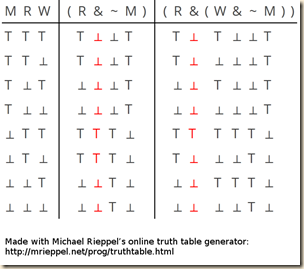As we can see, there are 8 rows and 13 columns, thus 104 Ts and Fs.

We turn now to properties held by singular propositions. Agler gives the following definitions for tautology, contradiction, and contingency for truth tree evaluations.

Tautology: A proposition ‘P’ is a tautology if and only if ‘P’ is true under every valuation. A truth tree shows that ‘P’ is a tautology if and only if a tree of the stack of ‘¬P’ determines a closed tree.

Contradiction: A proposition ‘P’ is a contradiction if and only if ‘P’ is false under every valuation. A truth tree shows that ‘P’ is a contradiction if and only if a tree of the stack of ‘P’ determines a closed tree.

Contingency: A proposition ‘P’ is a contingency if and only if ‘P’ is neither always false under every valuation nor always true under every valuation. A truth tree shows that ‘P’ is a contingency if and only if a tree of ‘P’ does not determine a closed tree and a tree of ‘¬P’ does not determine a closed tree.

(Agler 145)

So let us begin with tautology. As indicated above, we test a proposition to see if it is a tautology by making the tree for that proposition’s negation. If the tree is closed, that means there are no truth value assignments that can make the proposition’s negation true, which means that all truth value assignments will make the original proposition true. So let us consider the proposition: P∨¬P. We should thus place ¬(P∨¬P) as the first line of the tree.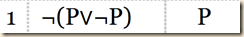Then we decompose this negated disjunction.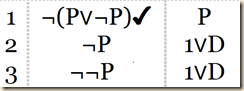[Note, in my version of the text, the justification shows the conjunction rule.] We then decompose the double negation.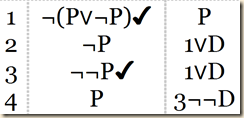As you can see, we have a formula and its negation along the branch, which means it is closed. The whole tree is thus closed. This means that all possible assignments make ¬(P∨¬P) false and therefore all assignments make P∨¬P true. It is thus a tautology.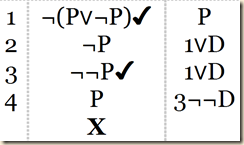Next we will test the proposition P→(Q∧¬P) to see if it is a tautology. We do this as you know by first placing its negation as the first line of the tree.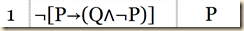We first decompose the negated conditional.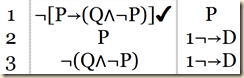Next we decompose the negated conjunction.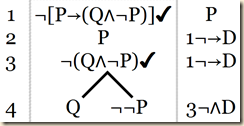There is no more to decompose on the left branch. It has no contradictions, so it is open.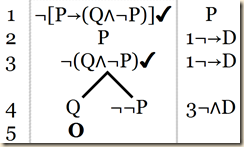At this point we do not need to go further, because we have determined that the tree is open. This means that the negation of P→(Q∧¬P) is not a contradiction, and therefore P→(Q∧¬P) is not a tautology. [Were we to continue the decomposition, we would decompose the double negation.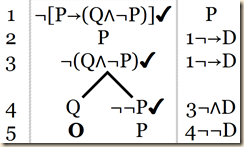And we would see that it is also open.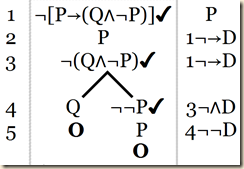] So we will need to conduct another test to see whether it is a contradiction or a contingency.

Agler first will show us a truth tree for a proposition that is a contradiction: P∧¬P.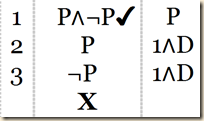Since there is only one branch and since it is closed, that means there is truth value assignment that can make P∧¬P true and thus it is a contradiction.

So now let us see if P→(Q∧¬P) is a contradiction or a contingency. So let us set is as the first line.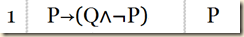We first decompose the conditional.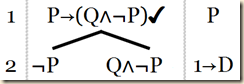For the the left branch, there is nothing more to decompose. Since there are no contradictions along this branch, we mark it as open.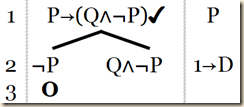Along the right branch we next decompose the conjunction.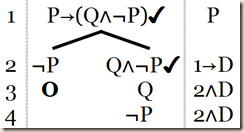We again have no more to do and also no contradictions, so we mark it as open.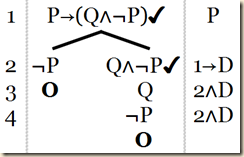This means that there are truth value assignments that make it true. Thus it is not a contradiction. And we already saw it was not a tautology. Thus it is a contingency. Here by the way is the truth table, which confirms this.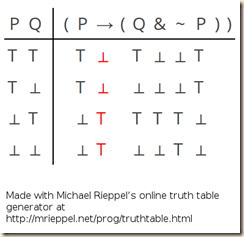So to test whether a proposition is a tautology, contradiction, or contingency, we do the following. [I will quote Agler after this. The following seems to be one set of tests, which begins with first by testing for tautology. However, the quoted material coming later might suggest you can begin first by testing for a contradiction.] First test the proposition to see if it is a tautology. We do this by seeing if the tree for the proposition’s negation has all closed branches.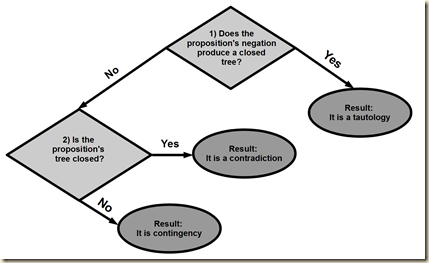If indeed they all close, then it is a tautology.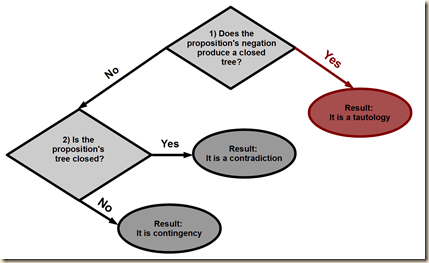If the proposition’s negation does not produce a closed tree, then the original proposition is either a contradiction or a contingency. So we make the tree for that original proposition.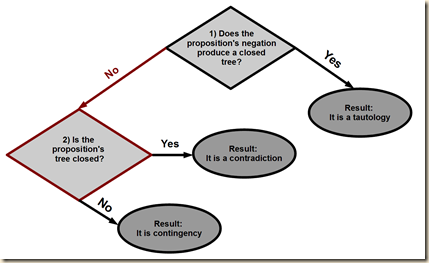If the proposition’s tree is closed, then it is a contradiction.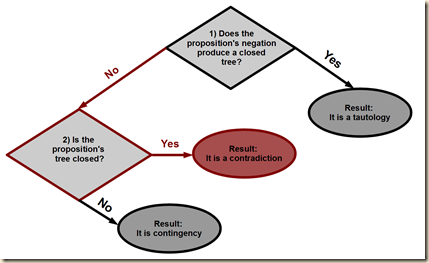But if it is an open tree, then it is a contingency [this is after we determined that it is not a tautology either.]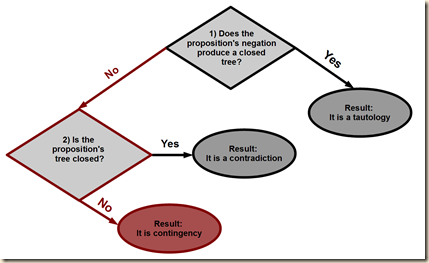To summarize, the truth-tree method can be used to determine whether a proposition ‘P’ is a tautology, contradiction, or contingency. In testing ‘P’ to see if it is a tautology, begin the tree with ‘¬P.’ If the tree closes, you know that it is a tautology. If the tree is open, then ‘P’ is either a contradiction or a contingency. Similarly, in testing ‘P’ to see if it is a contradiction, begin the tree with ‘P.’ If the tree closes, you know that it is a contradiction. If the tree is open, then ‘P’ is either a tautology or a contingency. Lastly, if the truth-tree test shows that ‘P’ is neither a contradiction nor a tautology, then ‘P’ is a contingency.

(Agler 147)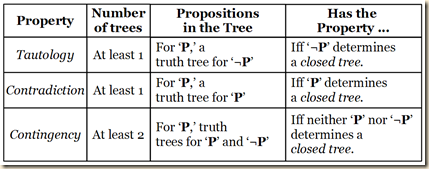4.6.4 Logical Equivalence

We just examined properties for singular propositions. We now examine equivalence, which is a property that can be held between just two propositions. Agler defines it in this way.

Equivalence: A pair of propositions ‘P,’ ‘Q’ is equivalent if and only if ‘P’ and ‘Q’ have identical truth values under every valuation. A truth tree shows that ‘P’ and ‘Q’ are equivalent if and only if a tree of ‘¬(PQ)’ determines a closed tree.

(147)

[Recall from above that we test a tautology of a proposition by seeing if its negation is a contradiction, that is, if it has a closed tree. If we find that a biconditional is a tautology, that means both sides of the biconditional will always have the same truth value. And were that the case, the propositions on both sides would be logically equivalent.] Agler further explains:

Two propositions ‘P’ and ‘Q’ are logically equivalent if and only if ‘P’ and ‘Q’ never have different truth values. It follows that if ‘P’ and ‘Q’ can never have different truth values, then ‘PQ’ is a tautology. Thus, in order to determine whether ‘P’ and ‘Q’ are logically equivalent, we only need to determine whether ‘PQ’ is a tautology. This is done by considering whether ‘¬(PQ)’ determines a closed tree.

(148)

We will examine an example, the propositions P∨¬P and ¬(P∧¬P). We next combine them biconditionally as (P∨¬P)↔¬(P∧¬P). We want to know if this is a tautology, so we negate it as ¬[(P∨¬P)↔¬(P∧¬P)], and we will see if its tree is closed. So first we set it as a proposition.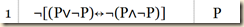Next we decompose the negated biconditional.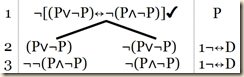Perhaps we see already a possible contradiction in the doubly negated formula in line 3. Were we to remove the double negation, we would have the conjunction of a proposition and its negation, which will close the branch. So first we decompose the double negation.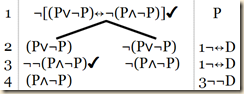Then we decompose the contradictory conjunction.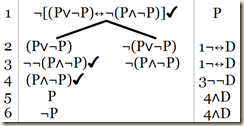And we then mark it as closed.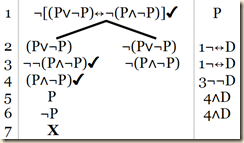Now moving to the other branch, we see that one proposition would be branching and the other stacking. So let us start with the stacking rule and decompose the negated disjunction.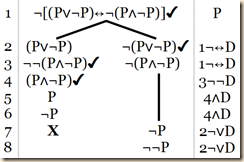As we can see, once we decompose the double negation, we will have a contradiction.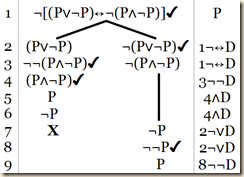So we mark this branch as closed.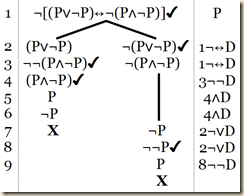That closes all branches. So this means that ¬[(P∨¬P)↔¬(P∧¬P)] is a contradiction. That furthermore means that (P∨¬P)↔¬(P∧¬P) is a tautology. Thus we can conclude that the propositions P∨¬P and ¬(P∧¬P) are logically equivalent.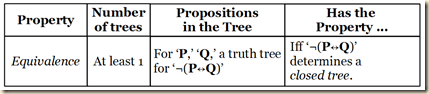4.6.5 Validity

Recall that an argument is valid “if and only if it is impossible for the premises to be true and the conclusion to be false” (149). The truth-table method for testing validity can involve making a very large table.

However, when we use the truth-tree method to test for validity, “the complexity of a truth tree is not a function of the number of propositional letters in the argument”  (149). [Since we want to know if it is impossible for the premises to be true and the conclusion false, we make a tree for the propositions with the conclusion negated. If the tree for that set of propositions is closed, that means there is no truth-assignment that can make the original set of premises and true and the conclusion false, and thus that argument is valid.]

Validity: An argument ‘P, Q, ... , Y Z’ is valid in PL if and only if it is impossible for the premises to be true and the conclusion false. A truth tree shows that an argument ‘P, Q, ... , Y Z’ is valid in PL if and only if ‘P,’ ‘Q,’ ‘R,’ ... , ‘Y,’ ‘¬Z’ determine a closed tree.

(Agler 149)

We first consider this example:

P→Q, P ⊢ Q

We need to convert it to a set of stacking propositions with a negated conclusion, which gives us: P→Q, P, and ¬Q. There is just one decomposition in this tree.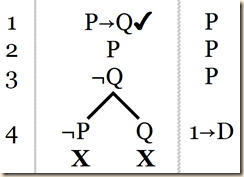Since the set of propositions P→Q, P, and ¬Q creates a closed tree, it is inconsistent. That means P→Q, P ⊢ Q is a valid argument (149).

Let us consider another example:

P→¬(Q→¬W), (Q→¬W)∨(Ρ↔S), P ⊢ S→P

We thus make a table for:

P→¬(Q→¬W), (Q→¬W)∨(Ρ↔S), P,¬(S→P)

[Since there are so many things to decompose, we should look for any contradictions that can be revealed quickly. We see that ¬(S→P) will make S and ¬P, which will contradict P. So we only have to make that one decomposition to determine it to be a closed tree.]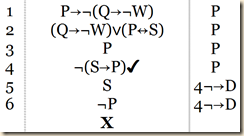As we can see, this creates a closed tree. Thus P→¬(Q→¬W), (Q→¬W)∨(Ρ↔S), P ⊢ S→P is valid.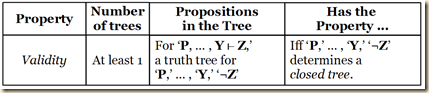(Agler 150)

In all, we have this table for truth-tree analysis for logical properties.(Agler 159)

Agler, David. Symbolic Logic: Syntax, Semantics, and Proof. New York: Rowman & Littlefield, 2013.

Michael Rieppel’s online truth table generator
http://mrieppel.net/prog/truthtable.html

.